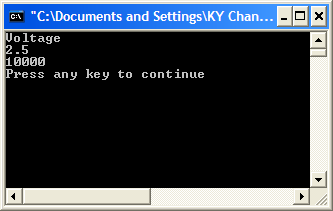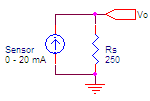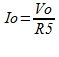The following information is for the EMANT300, EMANT380

# Exercise 4 – Console Input and Output

## Objective

• Learn Console Input

• Revise Console Output

• Revise Variables, Statements and Expressions

In the previous exercise, if we want to calculate the resistance given the voltage we need to edit the Visual Basic source code, recompile and rerun. It is easier if we can run the same program and interactively enter the voltage.

In this exercise, we will learn how to enter the voltage interactively using Console Input.

1.Open the Visual Basic solution VB_2010_Solution.sln.

2. Set the ConsoleIO project as the startup project.

3. View the project code by opening the project's Module1.vb

4. Press Ctrl+F5 to Start without Debugging to run your calculator program. Key in a voltage between 0 and 4.5. The program will return the resistance value.5. Press any key to return to the development environment.

6. Run the program several times. Enter a different value of voltage each time and find the resistance value.

## Program 4.1 Interactive Calculator

``````Module Module1
Sub Main()
Dim R1, Rs, Vcc, Vo As Double
Dim myinput As String
Console.WriteLine("Voltage")
Vo = Convert.ToDouble(myinput)
R1 = 10000
Vcc = 5
Rs = (R1 * Vo) / (Vcc - Vo)
Console.WriteLine(Rs)
End Sub
End Module
``````

## Data Type String

``````Dim myinput As String
``````

In the earlier exercise we have looked at the use of numeric variables. However, the processing of text data is also needed. Visual Basic provides the string data type and string variables can hold any characters. An example of a string is “Hello World”. If you want to assign a string variable, you must enclose the string in quotes

``````myinput = “Hello World”
``````

You can join or concatenate strings

``````myinput = “Hello” + “World”
``````

## Reading from Console

``````myinput = Console.ReadLine
``````

The ReadLine method of the Console class prompts the user for input and returns the string that was entered and assign it to the string variable myinput.

## Convert from string to double

``Vo = Convert.ToDouble(myinput)``

To convert the string variable to another data type, Visual Basic provides a Convert class in the System namespace that supports just about every conversion between intrinsic types, such as int, double and string. Our code converts the string to a double and assigns it to Vo.

The Convert class returns a type whose value is equivalent to the value of a specified type. Some of the common methods.

 Method Conversion ToBoolean Converts a specified value to an equivalent Boolean value. ToDateTime Converts a specified value to a DateTime. ToDouble Converts a specified value to a double-precision floating point number. ToInt32 Converts a specified value to a 32-bit signed integer. ToString Converts the specified value to its equivalent String representation. ToUInt32 Converts a specified value to a 32-bit unsigned integer.

## Measure current (optional exercise)In the process industry where sensors are located long distances from the measurement unit, the sensor is often connected to a signal conditioning unit which output a current that corresponds to the physical measurement.

A typical sensor could be a CO (carbon monoxide) sensor which outputs a 0 - 20 mA current that corresponds to a 0 – 300 ppm CO concentration. To measure this current, a simple solution would be to add a shunt resistance of 250 ohm.The following Visual Basic code calculates the current input given the voltage across the load resistance.

``````Dim R1, Io, Vo As Double
Dim myinput As String
Console.WriteLine("Voltage")